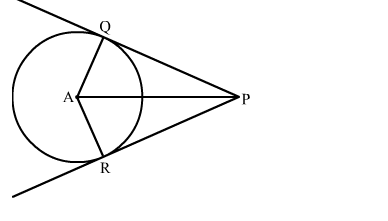# In the given figure, PQ and PR are tangents to a circle with centre A.`
Question:

In the given figure, PQ and PR are tangents to a circle with centre A. If ∠QPA = 27 then ∠QAR equals

(a) 63
(b) 117
(c) 126
(d) 153Solution:

We know that the radius and tangent are perperpendular at their point of contact
Now, In △PQA
∠PQA + ∠QAP + ∠APQ = 180            [Angle sum property of a triangle]
⇒ 90 + ∠QAP + 27 = 180                          [∵∠OAB = ∠OBA ]
⇒ ∠QAP = 63
In △PQA and △PRA
PQ = PR              (Tangents draw from same external point are equal)
QA = RA             (Radii of the circle)
AP = AP              (common)
By SSS congruency
△PQA ≅ △PRA
∠QAP = ∠RAP = 63
∴∠QAR = ∠QAP + ∠RAP = 63 + 63 = 126
Hence, the correct answer is option (c).